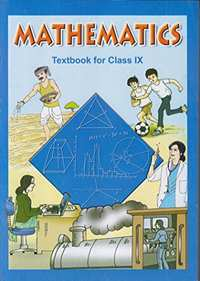Advertisement Remove all ads

# NCERT solutions for Class 9 Mathematics Textbook chapter 11 - Constructions [Latest edition]

#### ChaptersAdvertisement Remove all ads
Advertisement Remove all ads
Advertisement Remove all ads

## Chapter 11: Constructions

Exercise 11.1Exercise 11.2
Advertisement Remove all ads
Exercise 11.1[Page 191]

### NCERT solutions for Class 9 Mathematics Textbook Chapter 11 ConstructionsExercise 11.1[Page 191]

Exercise 11.1 | Q 1 | Page 191

Construct an angle of 90° at the initial point of a given ray and justify the construction.

Exercise 11.1 | Q 2 | Page 191

Construct an angle of 45° at the initial point of a given ray and justify the construction.

Exercise 11.1 | Q 3.1 | Page 191

Construct the angles of the following measurement:- 30°

Exercise 11.1 | Q 3.2 | Page 191

Construct the angles of the following measurement:- 22 1/2^@

Exercise 11.1 | Q 3.3 | Page 191

Construct the angles of the following measurement:- 15°

Exercise 11.1 | Q 4.1 | Page 191

Construct the 75° angles and verify by measuring it by a protractor.

Exercise 11.1 | Q 4.2 | Page 191

Construct the 105° angles and verify by measuring it by a protractor.

Exercise 11.1 | Q 4.3 | Page 191

Construct the 135° angles and verify by measuring it by a protractor.

Exercise 11.1 | Q 5 | Page 191

Construct an equilateral triangle, given its side and justify the construction.

Exercise 11.2[Page 195]

### NCERT solutions for Class 9 Mathematics Textbook Chapter 11 ConstructionsExercise 11.2[Page 195]

Exercise 11.2 | Q 1 | Page 195

Construct a triangle ABC in which BC = 7 cm, ∠B = 75° and AB + AC = 13 cm.

Exercise 11.2 | Q 2 | Page 195

Construct a triangle ABC in which BC = 8 cm, ∠B = 45° and AB − AC = 3.5 cm.

Exercise 11.2 | Q 3 | Page 195

Construct a triangle PQR in which QR = 6 cm, ∠Q = 60° and PR − PQ = 2 cm

Exercise 11.2 | Q 4 | Page 195

Construct a triangle XYZ in which ∠Y = 30°, ∠Z = 90° and XY + YZ + ZX = 11 cm.

Exercise 11.2 | Q 5 | Page 195

Construct a right triangle whose base is 12 cm and sum of its hypotenuse and other side is 18 cm.

## Chapter 11: Constructions

Exercise 11.1Exercise 11.2## NCERT solutions for Class 9 Mathematics Textbook chapter 11 - Constructions

NCERT solutions for Class 9 Mathematics Textbook chapter 11 (Constructions) include all questions with solution and detail explanation. This will clear students doubts about any question and improve application skills while preparing for board exams. The detailed, step-by-step solutions will help you understand the concepts better and clear your confusions, if any. Shaalaa.com has the CBSE Class 9 Mathematics Textbook solutions in a manner that help students grasp basic concepts better and faster.

Further, we at Shaalaa.com provide such solutions so that students can prepare for written exams. NCERT textbook solutions can be a core help for self-study and acts as a perfect self-help guidance for students.

Concepts covered in Class 9 Mathematics Textbook chapter 11 Constructions are Introduction of Constructions, Basic Constructions, Some Constructions of Triangles.

Using NCERT Class 9 solutions Constructions exercise by students are an easy way to prepare for the exams, as they involve solutions arranged chapter-wise also page wise. The questions involved in NCERT Solutions are important questions that can be asked in the final exam. Maximum students of CBSE Class 9 prefer NCERT Textbook Solutions to score more in exam.

Get the free view of chapter 11 Constructions Class 9 extra questions for Class 9 Mathematics Textbook and can use Shaalaa.com to keep it handy for your exam preparation

Advertisement Remove all ads
Share
Notifications

View all notifications

Forgot password?